# Hayes Process Macro - Model 1 - Moderation Analysis with Continuous Moderator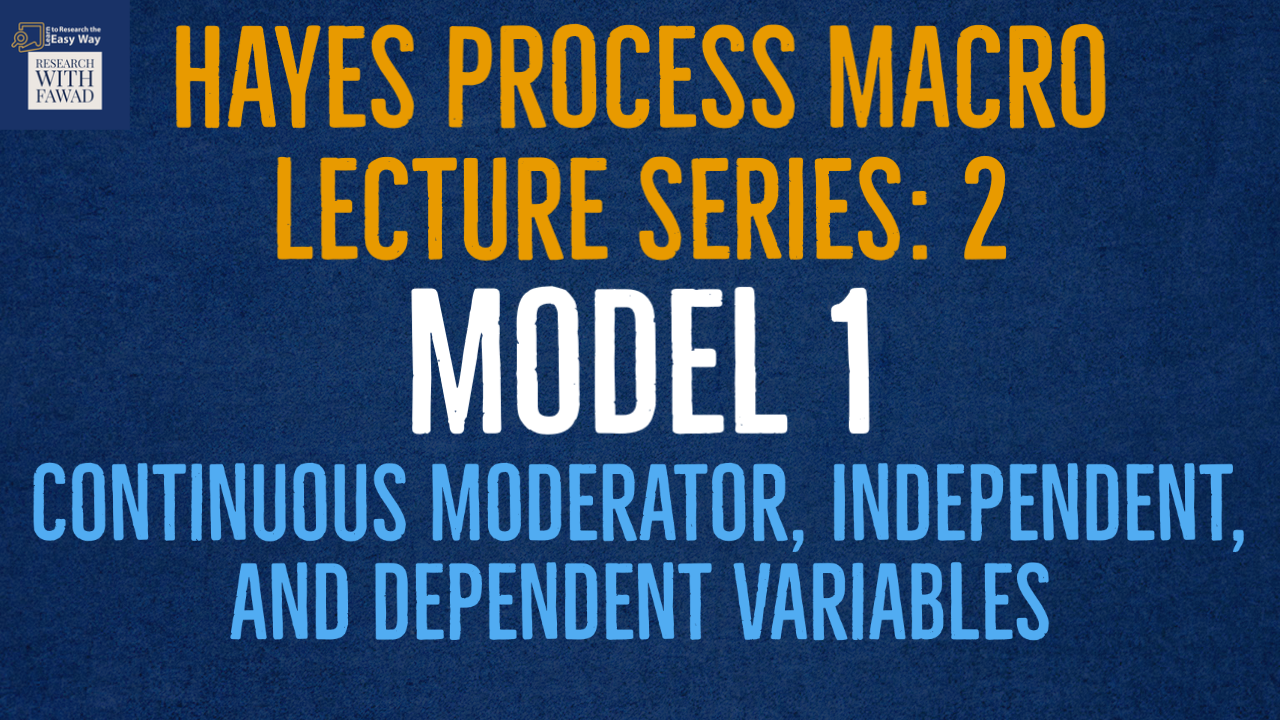### Hayes Process Macro - Lecture Series

The tutorial will guide on Model 1 of the Hayes Process Macro with Moderation Analysis using a Categorical Moderator, Continuous Independent, and Dependent Variables.

## Model Index

• All models have one primary IV and one DV. For the purposes of calculation of the indirect effects and conditional effects:
• The primary IV (variable X) is assumed to be continuous or dichotomous
• Moderators (variables W, V, Q, Z) are assumed to be continuous. Can be Categorical as well.
• Mediators (variable M, or M1, M2 etc.) are assumed to be continuous
• The DV (variable Y) is assumed to be continuous and to satisfy the assumptions of standard multiple regression. Can be Binary.

## Model 1: 1 Moderator [BASIC MODERATION] Continuous Moderator

• Example Variables: 1 predictor X, 1 moderator W, 1 outcome Y

Preliminary notes:

• Model 1 assumes that:
• The primary IV (variable X) is continuous or dichotomous
• Any moderators (variables W,V,Q,Z) are continuous, though the dichotomous moderators can be handled.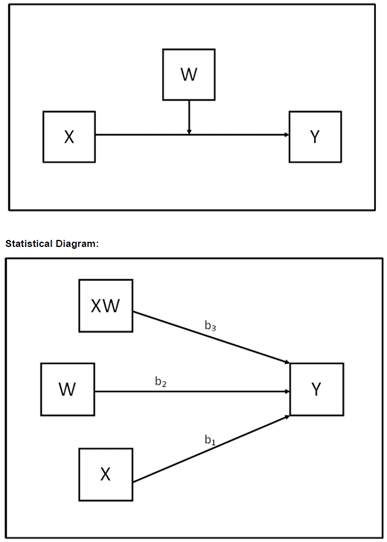## How to Run

• Step 1: Analyze -> Regression -> Process v4.0 by Andrew F. Hayes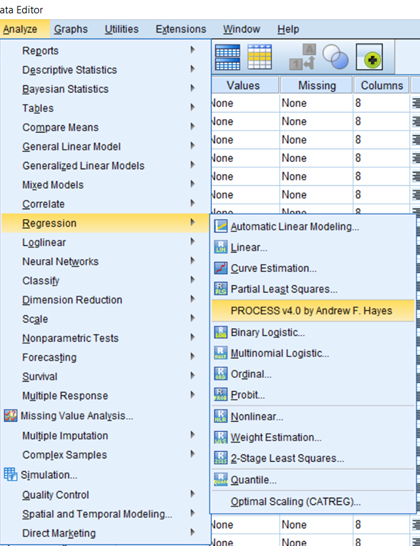Step 2: Put in the Required Variables. In this case,

• Y Variable (Dependent Variable) is OP
• X Variable (Independent Variable) is Culture
• Moderator Variable W: is Role Ambiguity (RA)
• Model Number is 1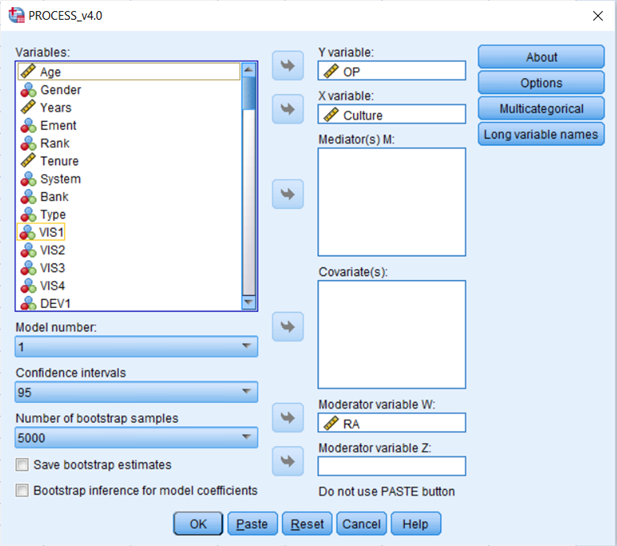• Step 3: Select Option Button, and Choose the options as shown in the figure below ()
• Select Generate code for visualizing interaction, this is to generate graphs for slope analysis
• From Mean center for construction of products groups box, select Only continuous variables that define products. This will mean center the variables the are part of the interaction term. We do mean centering to avoid multi-collinearity between predictor variables and the interaction term.
• From Moderation and conditioning, select -1SD, Mean, and +1SD, for Low, Average, and High level of Moderator.
• Finally, check Johnson-Neyman output, (Described Later in the tutorial).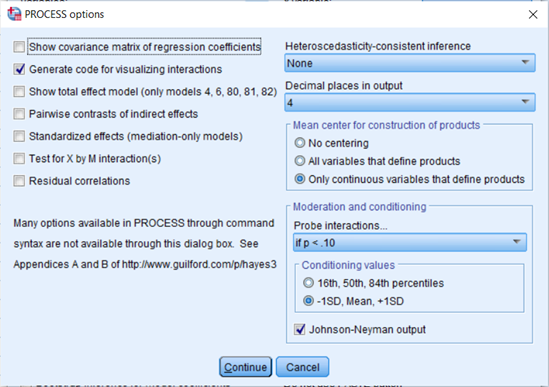## Output Interpretation - Basic Summary

• The first part of the output is the basic Description of Model along with the different variables where Y is Dependent Variable, X is Independent Variable, and W is the Moderating Variable.
• Model Summary, provides summary of the model with R, R-Sq, F Statistics, and P value for the overall model.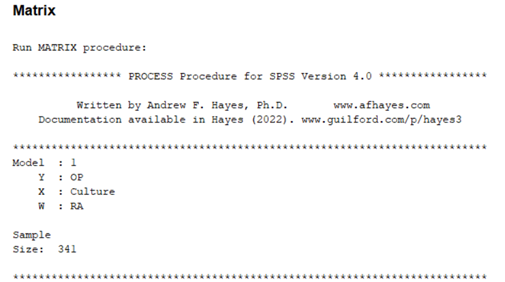## Output Interpretation - Model Summary

• Next, is the coefficients , with impact of Culture, RA, and the interaction effect to assess if there is moderation or not.
• In this example, since the interaction effect is significant, the Role Ambiguity moderates the relationship between Culture and OP
• Test of unconditional interaction, this shows the change in R-Sq due to interaction (x*w), this is also significant.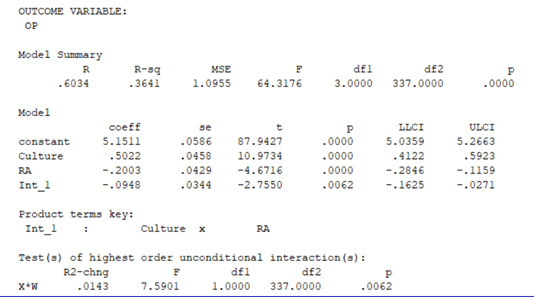## Johnson-Neyman intervals and simple slopes analysis

• Johnson-Neyman interval tells you the range of values of the moderator in which the slope of the predictor is significant vs. nonsignificant at a specified alpha level
• In simple terms Johnson-Neyman identifies regions in the range of the moderator variable where the effect of the focal predictor on the outcome is statistically significant and not significant.
• In this example, the impact of the moderating variable (RA), on the relationship between focal predictor (Collaborative Culture) and outcome (Organizational Performance) is significant till the value of RA is increased to 2.9281, if further increased there is no moderating effect of RA.